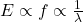## PLZ HELP ILL GIVE BRAINLIST! How does the energy of the different waves of the spectrum vary with wavelength ?

Question

PLZ HELP ILL GIVE BRAINLIST!
How does the energy of the different waves of the spectrum vary with wavelength ?

in progress 0
2 months 2021-07-29T18:22:07+00:00 1 Answers 2 views 0

The energy of a wave is inversely proportional to the wavelength of the wave.

As wavelength increases, the energy of the wave decreases.

As wavelength decreases, the energy of the wave increases.

Explanation:

The energy of a wave is directly proportional to the wave’s frequency. As frequency increases, so does the energy of the wave.(energy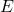is proportional to frequency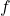)

How is this related to wavelength?

Frequency is inversely proportional to wavelength. That means that as frequency increases, wavelength decreases and as frequency decreases, wavelength increases.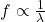(frequencyis inversely proportional to wavelength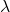)

Therefore, as wavelength increases, the energy of a wave decreases and as wavelength decreases, the energy of a wave increases.